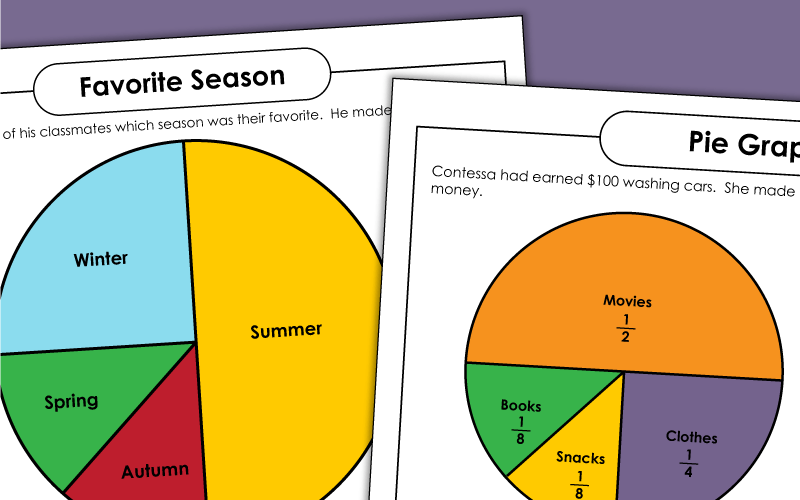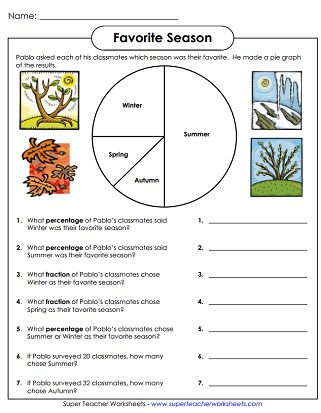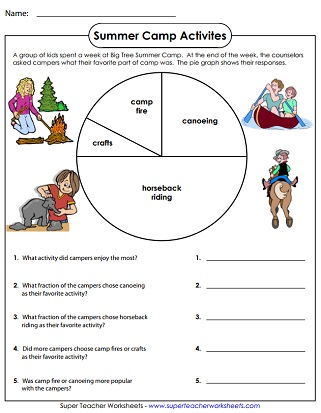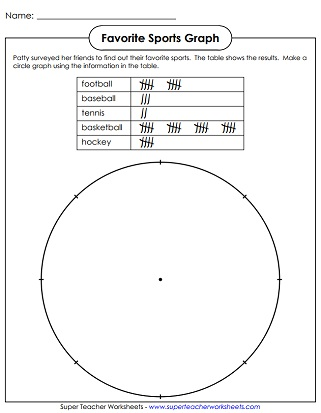# Pie Graphs (Circle Graphs)

This page has several pie graph worksheets. The "Basic Pie Graphs" require students to have a basic understanding of fractions. The "Advanced Pie Graphs" require students to understand percentages./p>## Pie Graphs: Basic(Fractions, No Precents)

Use the information in the summer camp pie graph to answer the questions. Requires knowledge of fractions.
Pie graph shows the various methods of transportation students used to get to school; Requires basic understanding of fractions

Pie graph shows students' field trip preferences; Requires knowledge of percentages and fractions
Pie graph shows students' favorite seasons; Requires knowledge of percentages and fractions
Pie graph shows how Contessa spends her money; Requires knowledge of percentages and fractions

Pictograph Worksheets

Bar Graph Worksheets

Printable bar graph worksheets with questions

Line Graph Worksheets

A collection basic, intermediate, and advanced of line graph worksheets

## Worksheet ImagesMy Account
Site Information